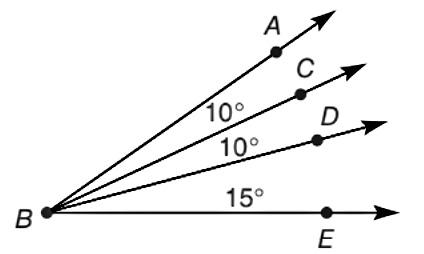Chapter 1.2, Problem 34E### Elementary Geometry for College St...

6th Edition
Daniel C. Alexander + 1 other
ISBN: 9781285195698

#### Solutions

Chapter
Section### Elementary Geometry for College St...

6th Edition
Daniel C. Alexander + 1 other
ISBN: 9781285195698
Textbook Problem
8 views

# An angle is bisected if its two parts have the same measure. Use three letters to name the angle that is bisected.To determine

To name:

The angle that is bisected, if its two parts have the same measure using three letters.

Explanation

Given,

An angle is bisected if its two parts have the same measure.

Since, the measure of angle formed between the sides BA, BC is equal to the measure of angle formed by the sides

### Still sussing out bartleby?

Check out a sample textbook solution.

See a sample solution

#### The Solution to Your Study Problems

Bartleby provides explanations to thousands of textbook problems written by our experts, many with advanced degrees!

Get Started